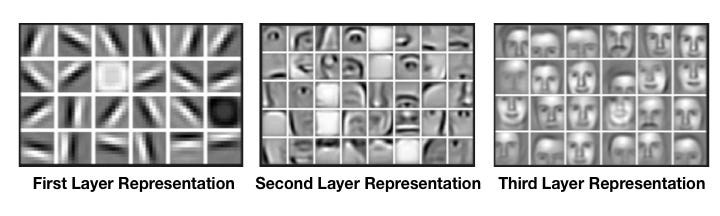Kernel sizes for multiple convolutional layer neural networks

In most examples I've seen of CNNs with multiple two or more convolutional layers the second layer has more kernels (feature masks) than the second layer, usually around twice as many. My intuition is telling me that the reason for this is that the first layer is picking out features from the input data and the second layer is picking out subsequent combinations of those features in the first convolutional layer. If there are 5 different possible features in the data then there are many more combinations of ways these features could show up in the data. If I'm not wrong there is $\sum\limits_{n=1}^{5}{5 \choose n}$ combinations. So why do we not have that many kernels in the second layer? Is it because of memory limitations, or is it because the number of kernels is more related to the number of possible classes?